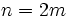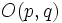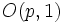# Category:Differential manifolds with reduced structure group

The smaller the structure group, the stronger the structure on the differential manifold. In other words, a differential manifold with a given structure group automatically has any bigger group containing that, as structure group. The important subgroups of the general linear group occurring here are:

•$U(n,\mathbb{C})$ which corresponds to almost Hermitian manifold
•$GL(n,\mathbb{C})$ which contains$U(n,\mathbb{C})$ and corresponds to almost complex manifold. Thus almost Hermitian manifold is a stronger structure than almost complex manifold.
•$Sp(2n,\mathbb{R})$ which contains$U(n,\mathbb{C})$ and corresponds to almost symplectic manifold. Thus almost Hermitian manifold is a stronger structure than almost symplectic manifold.
•$O(n,\R)$ which corresponds to Riemannian manifold. When$n=2m$, this contains$U(n,\mathbb{C})$. Thus, almost Hermitian manifold is a stronger structure than Riemannian manifold
•$GL^+(n,\R)$ which contains$Sp(2m,\R)$ when$n=2m$, and corresponds to oriented manifold. Thus oriented manifold is a stronger structure than almost symplectic manifold.

Lorentzian and pseudo-Riemannian manifolds correspond to pseudo-orthogonal groups$O(p,q)$ and$O(p,1)$.

## Pages in category "Differential manifolds with reduced structure group"

The following 7 pages are in this category, out of 7 total.